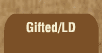Mathematics and Dyslexia

Tips for Learning the Times Tables

Tools for the Times Tables & Workbook

Home > Math > times tables practice > Threes (all of them)

## The Threes Times Tables (all of them)

You may only have to do this page once! If not, you might want to go back and practice the smaller groups that feature the ones you still aren't quick with.

1. 3 x 3=
2. 3 x 5=
3. 1 x 3=
4. 3 x 9=
5. 3 x 7=
6. 10 x 3=
7. 6 x 3=
8. 3 x 4=
9. 8 x 3=
10. 3 x 2=
11. 3 x 10=
12. 0 x 3=
13. 7 x 3=
14. 3 x 8=
15. 9 x 3=